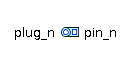Pressure Reducing Valve - MapleSim Help

Pressure Reducing Valve

Reducing valve with opening area linearly dependent on pressure differenceDescription

The Pressure Reducing Valve component models a hydraulic reducing valve as a sharp-edge orifice with the orifice area dependent on the pressure across the valve.

The area varies linearly from ${A}_{\mathrm{open}}$ to ${A}_{\mathrm{close}}$ as the pressure varies from ${p}_{\mathrm{contract}}$ to ${p}_{\mathrm{close}}$, and remains at the endpoints for pressures outside this range.

Based on the orifice area, the pressure vs flow rate relationships are derived by the formulation used in the Orifice component.Formulation Approaches Two approaches were taken for formulation of the flow equation inside the device. When the boolean parameter $\mathrm{Use constant Cd}$ is true, a constant coefficient of discharge (${C}_{d}$) is used, otherwise a variable coefficient of discharge with maximum value (${C}_{d\left(\mathrm{max}\right)}$) and a critical flow number (${\mathrm{Crit}}_{\mathrm{no}}$) are used.Optional Volumes The boolean parameters Use volume A and Use volume B, when true, add optional volumes ${V}_{A}$  and ${V}_{B}$ to ports A and B, respectively. See Port Volumes for details. If two orifices or valves are connected, enabling a volume at the common port reduces the stiffness of the system and improves the solvability.Equations $p={p}_{A}-{p}_{B}$ $\mathbf{Orifice Fluid Equations}$ $\left\{\begin{array}{cc}p=\frac{\mathrm{\pi }}{4}\frac{\mathrm{\rho }\mathrm{\nu }q}{{C}_{d}^{2}{A}_{\mathrm{cs}}\sqrt{\mathrm{\pi }{A}_{\mathrm{cs}}}}{\left(\frac{16{q}^{4}}{{\mathrm{\pi }}^{2}{A}_{\mathrm{cs}}^{2}{\mathrm{\nu }}^{4}}+{\mathrm{\Re }}_{\mathrm{Cr}}^{4}\right)}^{\frac{1}{4}}& \mathrm{Use constant Cd}=\mathrm{true}\\ q={C}_{d\left(\mathrm{max}\right)}\mathrm{tanh}\left(4\frac{\sqrt{\frac{{A}_{\mathrm{cs}}}{\mathrm{\pi }}\frac{2\left|p\right|}{\mathrm{\rho }}}}{\mathrm{\nu }{\mathrm{Crit}}_{\mathrm{no}}}\right){A}_{\mathrm{cs}}\sqrt{\frac{2\left|p\right|}{\mathrm{\rho }}}\mathrm{sign}\left(p\right)& \mathrm{otherwise}\end{array}$ $\mathbf{Orifice Area Equations}$ $\left\{\begin{array}{cc}{A}_{\mathrm{cs}}={A}_{i}={A}_{t}& \mathrm{Exact}=\mathrm{true}\\ \left\{{A}_{\mathrm{cs}}=\mathrm{min}\left({A}_{\mathrm{open}},\mathrm{max}\left({A}_{\mathrm{close}},{A}_{i}\right)\right),{t}_{c}\frac{\mathrm{d}{A}_{i}}{\mathrm{d}t}+{A}_{i}={A}_{t}\right\}& \mathrm{otherwise}\end{array}$ ${A}_{t}=\left\{\begin{array}{cc}{A}_{\mathrm{close}}& p\le {p}_{\mathrm{close}}\\ {A}_{\mathrm{close}}+\left(p-{p}_{\mathrm{close}}\right)\frac{{A}_{\mathrm{open}}-{A}_{\mathrm{close}}}{{p}_{\mathrm{open}}-{p}_{\mathrm{close}}}& p<{p}_{\mathrm{open}}\\ {A}_{\mathrm{open}}& \mathrm{otherwise}\end{array}$ $\mathbf{Optional Volume Equations}$ ${V}_{{f}_{A}}=\left\{\begin{array}{cc}\mathrm{Va}\left(1+\frac{{p}_{A}}{\mathrm{El}}\right)& \mathrm{Use volume A}=\mathrm{true}\\ 0& \mathrm{otherwise}\end{array}\phantom{\rule[-0.0ex]{5.0ex}{0.0ex}}{V}_{{f}_{B}}=\left\{\begin{array}{cc}\mathrm{Vb}\left(1+\frac{{p}_{B}}{\mathrm{El}}\right)& \mathrm{Use volume B}=\mathrm{true}\\ 0& \mathrm{otherwise}\end{array}$ $q={q}_{A}-{q}_{{V}_{A}}=-\left({q}_{B}-{q}_{{V}_{B}}\right)$ ${q}_{{V}_{A}}=\left\{\begin{array}{cc}\frac{\mathrm{d}{V}_{{f}_{A}}}{\mathrm{d}t}& \mathrm{Use volume A}=\mathrm{true}\\ 0& \mathrm{otherwise}\end{array}\phantom{\rule[-0.0ex]{4.0ex}{0.0ex}}{q}_{{V}_{B}}=\left\{\begin{array}{cc}\frac{\mathrm{d}{V}_{{f}_{B}}}{\mathrm{d}t}& \mathrm{Use volume B}=\mathrm{true}\\ 0& \mathrm{otherwise}\end{array}$Variables

 Name Units Description Modelica ID $p$ $\mathrm{Pa}$ Pressure drop from A to B p ${p}_{X}$ $\mathrm{Pa}$ Pressure at port X pX $q$ $\frac{{m}^{3}}{s}$ Flow rate from A to B q ${q}_{X}$ $\frac{{m}^{3}}{s}$ Flow into port X qX ${A}_{\mathrm{cs}}$ ${m}^{2}$ Cross-sectional area of orifice Acs ${A}_{i}$ ${m}^{2}$ Filtered interpolated area At ${A}_{t}$ ${m}^{2}$ Interpolated area At ${q}_{\mathrm{VX}}$ $\frac{{m}^{3}}{s}$ Flow rate into port X's optional volume qVX ${V}_{{f}_{X}}$ ${m}^{3}$ Effective volume at port X VfXConnections

 Name Description Modelica ID $\mathrm{portA}$ Upstream hydraulic port portA $\mathrm{portB}$ Downstream hydraulic port portBParametersGeneral

 Name Default Units Description Modelica ID ${p}_{\mathrm{close}}$ $2.1·{10}^{7}$ $\mathrm{Pa}$ Pressure at which valve is fully closed (A = Aclose) pclose ${p}_{\mathrm{contract}}$ $1.9·{10}^{7}$ $\mathrm{Pa}$ Pressure till which the valve is fully open (A = Aopen) pcontract ${A}_{\mathrm{close}}$ $1·{10}^{-12}$ ${m}^{2}$ Valve area when closed (leakage) Aclose ${A}_{\mathrm{open}}$ $1·{10}^{-5}$ ${m}^{2}$ Valve area when fully open Aopen $\mathrm{Exact}$ $\mathrm{false}$ When true (checked) a first-order dynamics is used for the valve area Exact ${t}_{c}$ $0.1$ $s$ Time constant used in first-order dynamics; used when $\mathrm{Exact}=\mathrm{true}$ tcOrifice

 Name Default Units Description Modelica ID $\mathrm{Use constant Cd}$ $\mathrm{true}$ True (checked) means a constant coefficient of discharge is implemented, otherwise a variable ${C}_{d}$ is used in flow calculation UseConstantCd ${C}_{d}$ $0.7$ Flow-discharge coefficient; used when $\mathrm{Use constant Cd}$ is true Cd ${\mathrm{\Re }}_{\mathrm{Cr}}$ $12$ Reynolds number at critical flow; used when $\mathrm{Use constant Cd}$ is true ReCr ${C}_{d\left(\mathrm{max}\right)}$ $0.7$ Maximum flow-discharge coefficient; used when $\mathrm{Use constant Cd}$ is false Cd_max ${\mathrm{Crit}}_{\mathrm{no}}$ $1000$ Critical flow number; used when $\mathrm{Use constant Cd}$ is false Crit_noOptional Volumes

 Name Default Units Description Modelica ID $\mathrm{Use volume A}$ $\mathrm{false}$ True (checked) means a hydraulic volume chamber is added to portA useVolumeA ${V}_{A}$ $1·{10}^{-6}$ ${m}^{3}$ Volume of chamber A Va $\mathrm{Use volume B}$ $\mathrm{false}$ True (checked) means a hydraulic volume chamber is added to portB useVolumeB ${V}_{B}$ $1·{10}^{-6}$ ${m}^{3}$ Volume of chamber B Vb